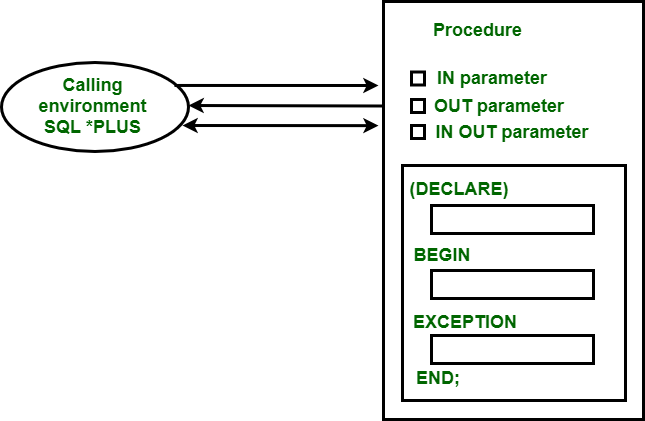Related Articles

# Argument Modes in PL/SQL

• Last Updated : 23 Oct, 2020

Argument modes are basically used to describe the behavior of the formal parameters. There are three types of argument modes which are used in the sub-program, which are as follows –

1. IN Mode
2. OUT Mode
3. IN OUT ModeRepresentation of how modes interact with calling environment

• IN Mode :
It is the default argument mode in subprogram. This mode passes a constant value from the calling environment into the subprogram.

Example –
The following example illustrates the working of IN Mode argument –

```SQL> CREATE OR REPLACE PROCEDURE PR1(X IN NUMBER, Y IN NUMBER)
S NUMBER;
BEGIN
S:=X+Y;
DBMS_OUTPUT.PUT_LINE('SUM IS : '||S);
END PR1;```

Output –

`Procedure created.`
```SQL> DECLARE
N1 NUMBER:=10;
N2 NUMBER:=20;
BEGIN
PR1(N1, N2);
END;```

Output –

```SUM IS : 30
PL/SQL procedure successfully completed.
SQL>```

• OUT Mode :
This mode passes a value from the subprogram to the calling environment.

Example –
The following example illustrates the working of OUT Mode argument –

```SQL> CREATE OR REPLACE PROCEDURE PR2(2 OUT NUMBER) AS
X NUMBER:=11;
Y NUMBER:=22;
BEGIN
Z:=X+Y;
END;```

Output –

`Procedure created.`
```SQL> DECLARE
R NUMBER;
BEGIN
PR2(R);
DBMS_OUTPUT.PUT_LINE('RESULT IS: '||R);
END;```

Output –

```RESULT IS : 33
PL/SQL procedure successfully completed.
SQL>```

• IN OUT Mode :
This mode is a mixture of both IN n=and OUT mode. Just like IN mode it passes a value from the calling environment in subprogram and like a OUT mode it possibly pass different value from the subprogram back to the calling environment using the same parameter.

Example –
The following example illustrates the working of OUT Mode argument –

```SQL> CREATE OR REPLACE PROCEDURE PR3(2 OUT NUMBER) AS
A NUMBER:=11;
BEGIN
B:=A+B;
END;```

Output –

`Procedure created.`
```SQL> DECLARE
R NUMBER:=22;
BEGIN
PR3(R);
DBMS_OUTPUT.PUT_LINE('RESULT IS: '||R);
END;```

Output –

```RESULT IS : 33
PL/SQL procedure successfully completed.
SQL>```

Attention reader! Don’t stop learning now. Learn SQL for interviews using SQL Course  by GeeksforGeeks.

My Personal Notes arrow_drop_up